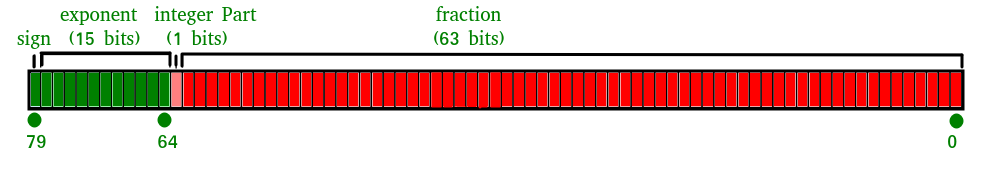Open In App

# JavaScript Number isSafeInteger() Method

What is a Safe Integer?
A safe integer is an integer that has the following properties

• A number that can be represented as an IEEE-754 double precision number i.e. all integers from (253 – 1) to -(253 – 1)).

What is an IEEE-754 double-precision number?
Double-precision floating-point format is a computer number format, which occupies 64 bits in computer memory. It represents a wide range of numeric values by using a floating-point.

The IEEE 754 standard specifies a binary64 as having:

• Sign bit: 1 bit
• Exponent: 11 bits
• Significant precision: 53 bits (52 explicitly stored)isSafeInteger() Method In JavaScript: The isSafeInteger() method in JavaScript is used to check whether a number is a safe integer or not.

Syntax:

`Number.isSafeInteger(Value)`

Parameters:

• Value: It is the number to be checked for safeinteger() method.

Return Value: The toExponential() method in JavaScript returns true if the value is a safe integer Number, else it returns false.

Below are examples of the Number isSafeInteger() Method.

Example 1: This example uses the safeinteger() method to check if 4 is a safe integer or not.

## Javascript

 `console.log(``"Output : "` `+ Number.isSafeInteger(44));`

Output

```Output : true

```

Example 2: Passing a positive number as an argument in the isSafeInteger() method.

## Javascript

 `console.log(``"Output : "` `+ Number.isSafeInteger(23));`

Output

```Output : true

```

Example 3: Passing a negative number as an argument in the isSafeInteger() method.

## Javascript

 `console.log(``"Output : "` `+ Number.isSafeInteger(-23));`

Output

```Output : true

```

Example 4: Passing a number(with decimals) as an argument in the isSafeInteger() method.

## Javascript

 `console.log(``"Output : "` `+ Number.isSafeInteger(0.5));`

Output

```Output : false

```

Example 5: Passing an equation( which equates to an infinite value) as an argument in the isSafeInteger() method.

## Javascript

 `console.log(``"Output : "` `+ Number.isSafeInteger(0 / 0));`

Output

```Output : false

```

Code Explanation: JavaScript uses double-precision floating-point format numbers as specified in IEEE 754 and can only safely represent numbers between -(253 – 1) and 253 – 1. If the parameter passed lies in this specified range then the number.isSafeInteger() method returns true else false.

We have a complete list of Javascript Number Methods, to check those please go through the Javascript Number Complete Reference article.

## Supported Browsers:

• Google Chrome 34 and above
• Firefox 32 and above
• Apple Safari 10 and above
• Opera 21 and above
• Edge 12 and above